In :
%load_ext autoreload

In :
autoreload 2

In :
%matplotlib inline

In :
import matplotlib.pyplot as plt
import numpy as np
import seaborn as sn

import pycollocation


## Example: Solow model with Cobb-Douglas production

The Solow model is a model of economic growth as a process of physical capital accumulation. By far the most common version of the Solow model assumes Cobb-Douglas functional form for intensive output:

$$f(k) = k^{\alpha}.$$
In :
def cobb_douglas_output(k, alpha, **params):
return k**alpha


After a bit of algebra, the Solow model with Cobb-Douglas production can be reduced down to a single non-linear ordinary differential equation (ODE) and an initial condition for capital (per unit effective labor supply)...

$$\dot{k}(t) = s k(t)^{\alpha} - (g + n + \delta) k(t),\ k(0) = k_0$$

...the above equation says that the rate of change of the stock of physical capital (per unit effective labor supply), $\dot{k}(t)$, is the difference between the actual level of investment in physical capital, $sk(t)^{\alpha}$, and the amount of investment required to maintain the current level of physical capital, $(g + n + \delta) k(t)$.

In :
def standard_solow_model(t, k, alpha, delta, g, n, s, **params):
return [s * cobb_douglas_output(k, alpha) - (g + n + delta) * k]

def initial_condition(t, k, k0, **params):
return [k - k0]


To complete the model we need to define some parameter values.

In :
params = {'g': 0.02, 's': 0.1, 'n': 0.02, 'alpha': 0.15, 'delta': 0.04, 'k0': 1.0}


## Solving the model with pyCollocation

### Defining a pycollocation.IVP instance

In :
pycollocation.problems.IVP?

In :
standard_solow_ivp = pycollocation.problems.IVP(bcs_lower=initial_condition,
number_bcs_lower=1,
number_odes=1,
params=params,
rhs=standard_solow_model,
)


### Finding a good initial guess for $k(t)$¶

Theory tells us that, starting from some initial condition $k_0$, the solution to the Solow model converges monotonically toward its long run equilibrium value $k^*$. Our initial guess for the solution should preserve this property...

In :
def equilibrium_capital(alpha, delta, g, n, s, **params):
"""Equilibrium value of capital (per unit effective labor supply)."""
return (s / (g + n + delta))**(1 / (1 - alpha))

In :
def initial_mesh(t, T, num, problem):
ts = np.linspace(t, T, num=num)
kstar = equilibrium_capital(**problem.params)
ks = kstar - (kstar - params['k0']) * np.exp(-ts)
return ts, ks


### Solving the model¶

In :
pycollocation.solvers.Solver?


### Orthogonal polynomial basis functions

In :
# Choose your basis functions and create a solver
polynomial_basis = pycollocation.basis_functions.PolynomialBasis()
solver = pycollocation.solvers.Solver(polynomial_basis)

# compute the initial mesh
boundary_points = (0, 100)
ts, ks = initial_mesh(*boundary_points, num=1000, problem=standard_solow_ivp)

# compute the initial coefs guess
basis_kwargs = {'kind': 'Chebyshev', 'domain': boundary_points, 'degree': 15}
k_poly = polynomial_basis.fit(ts, ks, **basis_kwargs)
initial_coefs = k_poly.coef

# specify the collocation nodes
nodes = polynomial_basis.roots(**basis_kwargs)

# solve the model!
solution = solver.solve(basis_kwargs, boundary_points, initial_coefs,
nodes, standard_solow_ivp)

In :
k_soln, = solution.evaluate_solution(ts)
plt.plot(ts, k_soln)
plt.show()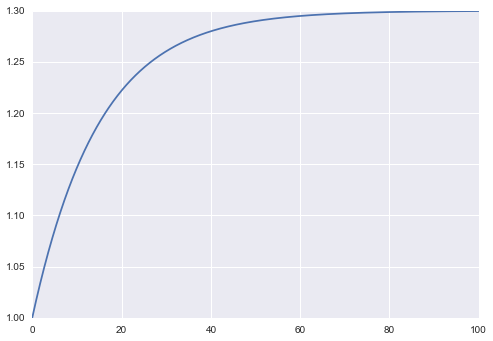In :
k_resids, = solution.evaluate_residual(ts)
plt.plot(ts, k_resids)
plt.show()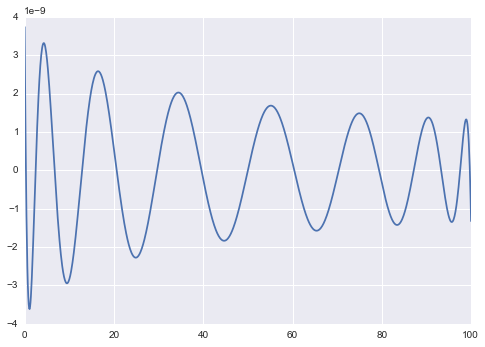In :
k_normalized_resids, = solution.normalize_residuals(ts)
plt.plot(ts, np.abs(k_normalized_resids))
plt.yscale('log')
plt.show()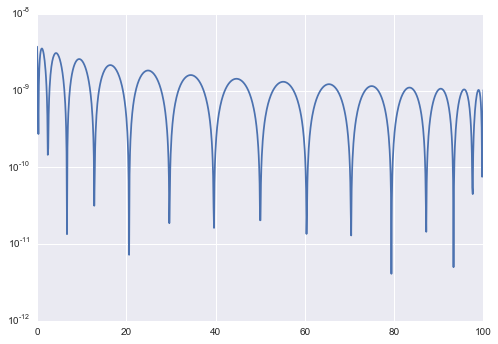### B-spline basis functions

In :
bspline_basis = pycollocation.basis_functions.BSplineBasis()
solver = pycollocation.solvers.Solver(bspline_basis)

ts, ks = initial_mesh(*boundary_points, num=250, problem=standard_solow_ivp)

tck, u = bspline_basis.fit([ks], u=ts, k=5, s=0)
knots, coefs, k = tck
initial_coefs = np.hstack(coefs)

basis_kwargs = {'knots': knots, 'degree': k, 'ext': 2}
nodes = np.linspace(*boundary_points, num=249)
solution = solver.solve(basis_kwargs, boundary_points, initial_coefs,
nodes, standard_solow_ivp)

In :
solution.result.success

Out:
True
In :
k_soln, = solution.evaluate_solution(ts)
plt.plot(ts, k_soln)
plt.show()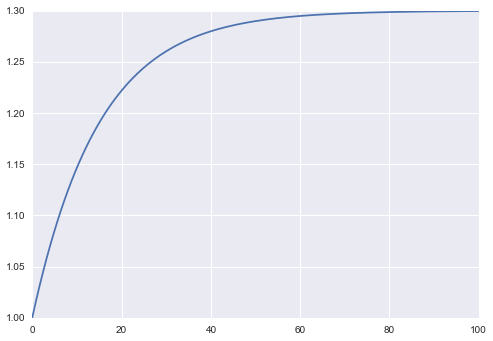In :
k_resids, = solution.evaluate_residual(ts)
plt.plot(ts, k_resids)
plt.show()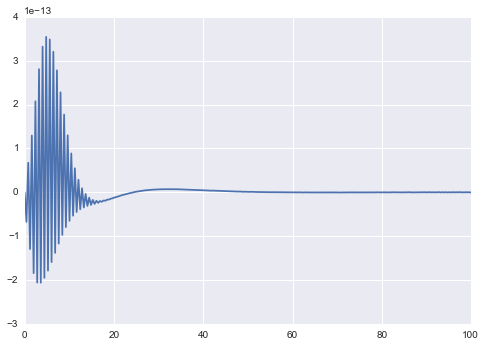In :
k_normalized_resids, = solution.normalize_residuals(ts)
plt.plot(ts, np.abs(k_normalized_resids))
plt.yscale('log')
plt.show()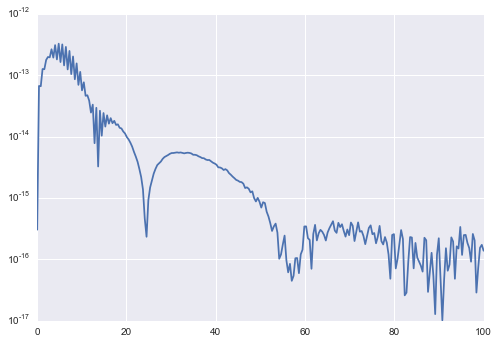# Example: Generic Solow model of economic growth

Can we refactor the code above so that it can be solve a Solow model for arbitrary intensive production $f$? Yes!

In :
from pycollocation.tests import models


Example usage...

In :
def ces_output(k, alpha, sigma, **params):
rho = (sigma - 1) / sigma
if rho == 0:
y = cobb_douglas_output(k, alpha)
else:
y = (alpha * k**rho + (1 - alpha))**(1 / rho)
return y

def ces_equilibrium_capital(g, n, s, alpha, delta, sigma, **params):
"""Steady state value for capital stock (per unit effective labor)."""
rho = (sigma - 1) / sigma
if rho == 0:
kss = (s / (g + n + delta))**(1 / (1 - alpha))
else:
kss = ((1 - alpha) / (((g + n + delta) / s)**rho - alpha))**(1 / rho)
return kss

ces_params = {'g': 0.02, 's': 0.1, 'n': 0.02, 'alpha': 0.15, 'delta': 0.04,
'sigma': 0.05, 'k0': 1.0}

In :
generic_solow_ivp = models.SolowModel(ces_output,
ces_equilibrium_capital,
ces_params)

In :
polynomial_basis = pycollocation.basis_functions.PolynomialBasis()
solver = pycollocation.solvers.Solver(polynomial_basis)

basis_kwargs = {'kind': 'Chebyshev', 'domain': [0, 100], 'degree': 15}

ts, ks = initial_mesh(basis_kwargs['domain'], 1000, standard_solow_ivp)
k_poly = polynomial_basis.fit(ts, ks, **basis_kwargs)
initial_coefs = k_poly.coef

solution = solver.solve(basis_kwargs, initial_coefs, standard_solow_ivp)

In :
k_soln, = solution.evaluate_solution(ts)
plt.plot(ts, k_soln)
plt.show()In :
k_normalized_resids, = solution.normalize_residuals(ts)
plt.plot(ts, np.abs(k_normalized_resids))
plt.yscale('log')
plt.show()In [ ]: